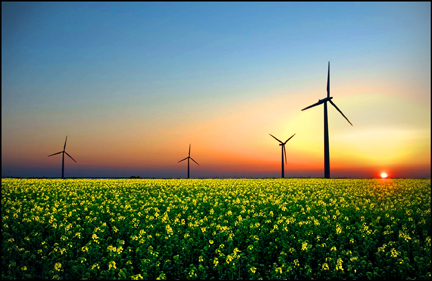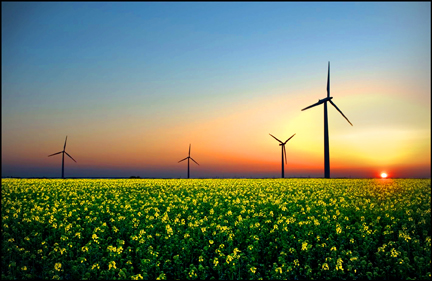# What is the Energy of Motion

We usually think of motion when an object is moving at a certain speed. We use a clock or other instrument to measure the speed of the object’s motion and then say it has kinetic energy. Does the universe have some kind of energy that gives motion to things? What is the energy of motion? What causes planets and light to move in space and time?## The Motion of the Universe

The Big Bang event moved energy into the universe at the speed of light and in that speed was the energy of motion.

When the energy arrived into the 3rd dimension the original energy was transformed into photon radiation that created particles of electrons, quarks and gluons.

During this conversion of energy into matter, the force of gravity and the strong nuclear force arrived.

Most people agree that this was the beginning of time but it was really the beginning of motion.

We have overlooked the fact that there is a constant energy that moves the universe.

We have taken this motion for granted as something that happens when energy is present.

What gives an electron its energy field? What makes light move out from an electron at the speed of light.

What makes particles of matter attract each other to make stars? Where does this energy of motion come from?

In the beginning, there was only one kind of motion. The motion of photon radiation moving at a high frequency.

When matter formed, the energy of motion was inside matter. Electrons move in a cloud of energy around a small particle.

Electrons become part of atoms that have a force of gravity and photons come out of that motion with the speed of light.

## Where does this Motion come from?

Energy has the potential to do work but where does the energy of motion come from? Follow me on this idea for a while.

Energy came into the universe and it went to work creating electrons, quarks and gluons.

Then these particles have energy that creates atoms.

But where does the energy of motion come from? Energy can not be created or destroyed but it can be converted into other things.

Energy steps down into mass and mass creates energy.

Said in another way, energy works to create mass, mass creates energy and energy creates mass.

Mass doesn’t create more mass or energy doesn’t create more energy.

For example, energy creates mass that is electrons, electrons create energy by some kind of force and that energy creates photons, then photons have energy etc.

If you are not confused yet, my question is where does the energy come from that provides the motion for atoms, electrons and photons?

Motion is what makes atoms vibrate with a frequency, and allows photons to travel at light speed.

It seems to me that frequencies of light come from the matter that created the light and that the frequency of atoms gives electrons the frequency that gets transferred to the photons of light.

My thinking is that frequencies are the source of energy that powers the universe.

Now that we have frequencies we have motion but where is the source of frequency?

Nikola Tesla said, “ To understand the universe think in terms of energy, frequency, and vibration.” and he said, “The day science starts to study non-physical phenomena, it will make more progress in one decade than in all the previous centuries of existence.”

## The Frequency of the Universe

Dimensions are the tools used to create a universe. Erik Lovin 2018

To understand the universe you must think outside the box of the 3rd dimension.

Look at the big picture and see that the energy that arrived into the 3rd dimension came from another place, realm or dimension.

The source of this energy must have had a very high frequency and it was stepped down into a lower frequency that came into the 3rd dimension.

If this frequency is in the space of the universe then spacetime is a physical thing that controls the motion in the universe.

The energy of motion is also called kinetic energy and the different types of energy include;  thermal energy, radiant energy, chemical energy, nuclear energy, electrical energy, motion energy, sound energy, elastic energy and gravitational energy.

But where is the frequency that moves electrons, quarks and gluons?

The frequency of the universe is in space itself and it allows photons to move at the speed of light.

Earth also has a frequency called the Schumann resonance which is 7.83 Hz.

I am still working to find the mechanism that works to provide the frequency to atoms and electrons.

My thought is that the mass of particles contains the number of quarks that gives the frequency to atoms or molecules.

Also, the mass governs the quantum gravity of particles which selects the proper frequency required.

This makes sense because the motion of particles is governed by gravity and or frequencies.

## Quantum and Classic Energy

Gravity can work in the quantum realm the same way as on the classic scale.

In the quantum fluctuations of atoms, the quantum chromodynamics (QCD) between quarks and gluons creates quantum gravity.

In the large scale of planets, gravity comes from the mass of the planet which is just the total amount of atoms times the quantum gravity of one atom.

Therefore, the gravity that causes motion comes from the frequency of spacetime that gives the quantum energy of motion to the QCD of quarks and gluons.

My conclusion is that the energy of motion comes from the frequency that arrived in the universe during the Big bang event.

We call this energy time but the proper term is energy of motion.